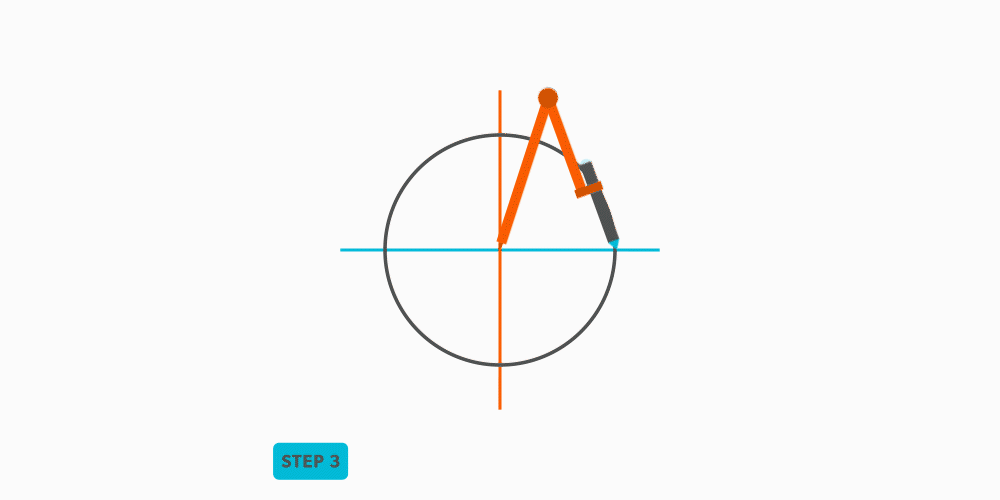Geometric Construction

# 9 Divide a Circle Into 12 Equal Parts

1. Draw an x-y axis.2. Draw a circle.3. From each point, swing the in both directions to cross the . (Remember, when we swing the along an of equal distance, we create 60°.) As we do it both ways, we end up with 30°-60°-90° all the way around the circumference.This is one of the most common techniques we use. Know it well!!!